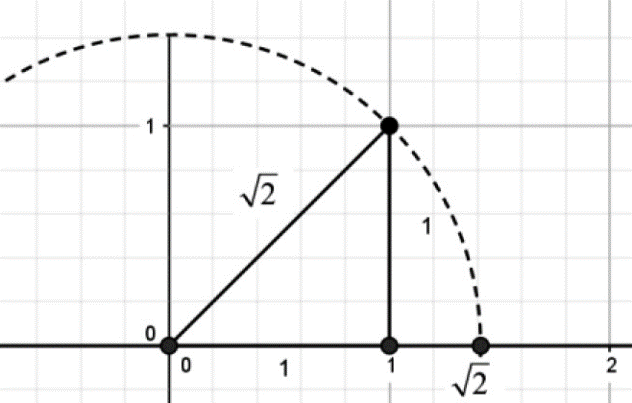# How many points are inside the hole revealed by the Dedekind cut?

It is illustrated by the figure that the set of the rational numbers is not a continuum, there are holes in it,One question is how many points are inside the hole?

From a geometrical perspective, I think treating real numbers as shorthands of Dedekind cuts of the set of rational numbers cannot convincingly answer the question - how many points are inside the hole revealed by Dedekind cut not produced by a rational number like $\begin{matrix} L & \ = \{ x \mid x \in \mathbb{Q},x \leq 0\} \cup \left\{ x \mid x \in \mathbb{Q},x > 0,x^{2} < 2 \right\} \\ U & \ = \mathbb{Q} - L = \left\{ x \mid x \in \mathbb{Q},x > 0,x^{2} > 2 \right\} \\ \end{matrix}$ ?

Every such Dedekind cut can be conceivably illustrated on the number axis, without a sound proof, to say such a cut corresponds with one and only one point on the number axis is far from trust , however, which is what exactly required by Cantor-Dedekind axiom  ,  . Unless a convincing proof is given, otherwise it is hard to acknowledge it is an obvious fact.

To swallow such fact and assume there are two points in the hole, and then get contradiction can only suggest it seems ok to claim the correspondence. In detail, the reasoning is as follows:

1. For definiteness we agree once for all, when speaking of the cut defining the rational number $r$ , to introduce this number into the upper class.

2. A fact between two Dedekind cuts(the density of $Q$ in $R$ ): For any pair of real numbers $\alpha$ and $\beta$ , where $\alpha > \beta$ , there can always be found a real, and even in particular a rational, number $r$ which lies between them, i.e. $\alpha > r > \beta$ (and, consequently, an infinite set of such rational numbers).

Since $\alpha > \beta$ the lower class $A$ of the cut defining the number $\alpha$ wholly contains the lower class $B$ for the number $\beta$ and it is not identical with $B$ . Hence a rational number $r$ can be found in $A$ which does not belong to $B$ .

1. We see the density of $\mathbf{Q}$ in $\mathbf{R}$ is an inherent or built-in property of the Dedekind cuts rather than a natural one, which is somewhat artificial . It is with the help of this property that the proponents of Dedekind cuts argue that " if we agree on the one to one correspondence between points on the number axis and the Dedekind cuts , then there is one and only one point in the hole revealed by Dedekind cut not produced by a rational number. Otherwise, there is one rational number $r$ which lies between them, i.e. $\alpha > r > \beta$ , which contradicts the fact $L \cup U = Q$ ".

But how do they convince people the rationality of the one to one correspondence? The question of how many points are inside the hole should not be portrayed as a result of the assumption, but should be a fact in itself .

1. Hans Niels Jahnke，A History of Analysis，p306 ↩︎

2. S. C. Malik， Principles of Real Analysis，P18; Editors: Ehrlich, p. (Ed.)，Real Numbers, Generalizations of the Reals, and Theories of Continua，page viii ↩︎